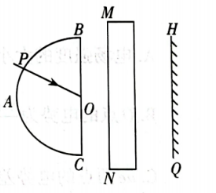$\text{A.}$ 在 $P$ 点时, 若将光屏稍向右平移, 光屏上红色与紫色光斑的间 距将增大 $\text{B.}$ 在 $P$ 点时, 光屏上红色光斑在紫色光斑的下方 $\text{C.}$ 在 $P$ 点时, 若撤除长方体玻璃砖, 光屏上红色光斑将向下移动 $\text{D.}$ 从 $B$ 移到 $C$ 的过程中, 光屏上红色光斑移动的平均速率比紫色光斑的大